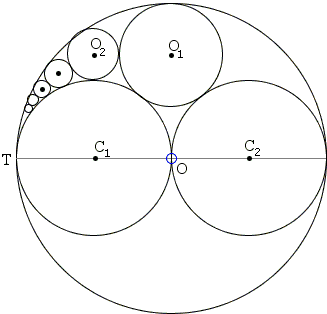# Pappus' Sangaku

Few sangaku illustrate better the disparity of the independent development of mathematics during the Edo period (1603-1867) of self-imposed seclusion of Japan from the Western world than those related to arbelos and Pappus' chain of circles. The tool of inversion so useful in solving problems involving circles remained unkown to the Japanese until late 19 century.

Consider the sangaku 1.8.2 from the collection by Fukagawa and Pedoe:

Circles C1(r) and C2(r) touch at O in the circle O(2r) and also touch O(2r), C1(r) touching at T. O1(r1) touches O(2r) internally and both C1(r) and C2(r) externally, O2(r2) touches O1(r1) and C1(r) externally and O(2r) internally, and so on. Find rn.Solution### References

1. H. Fukagawa, D. Pedoe, Japanese Temple Geometry Problems, The Charles Babbage Research Center, Winnipeg, 1989

Write to:

Charles Babbage Research Center
P.O. Box 272, St. Norbert Postal Station
Winnipeg, MBCircles C1(r) and C2(r) touch at O in the circle O(2r) and also touch O(2r), C1(r) touching at T. O1(r1) touches O(2r) internally and both C1(r) and C2(r) externally, O2(r2) touches O1(r1) and C1(r) externally and O(2r) internally, and so on. Find rn.With the help of inversion the solution is immediate. In the generalization of Archimedes' formula

rn = rk / (k²n² + k + 1).

where k is the ratio between the radii of the two inner circles, i.e. k = 1 in this case. Thus the answer to the problem is

rn = r / (n² + 2),

exactly the expression that appears in the Gothic Arc diagram.

In the very first volume (1826) of Crelle's Journal, J. Steiner proved several theorem concerning arbelos, the above being one of them. The sangaku however is dated 1801 (and was written in the Mie prefecture.)## Sangaku